# RD Sharma Solutions For Class 7 Maths Exercise 4.5 Chapter 4 Rational Numbers

RD Sharma Solutions for Class 7 Exercise 4.5 of Chapter 4 Rational Numbers in simple PDF are provide here. The main objective of providing exercise-wise solutions in PDF is to help students speed up their exam preparation. The solutions are designed based on the latest CBSE syllabus and exam pattern. These solutions are formulated by subject experts at BYJU’S in a unique manner to clear the doubts of students. In this exercise, students will thorough about equality of rational numbers. Two rational numbers said to be equivalent if one can be obtained from the other either by multiplying or by dividing its numerator and denominator by the same non-zero integer. To know more access to RD Sharma Solutions for Class 7.

## Download the PDF of RD Sharma Solutions For Class 7 Maths Chapter 4 – Rational Numbers Exercise 4.5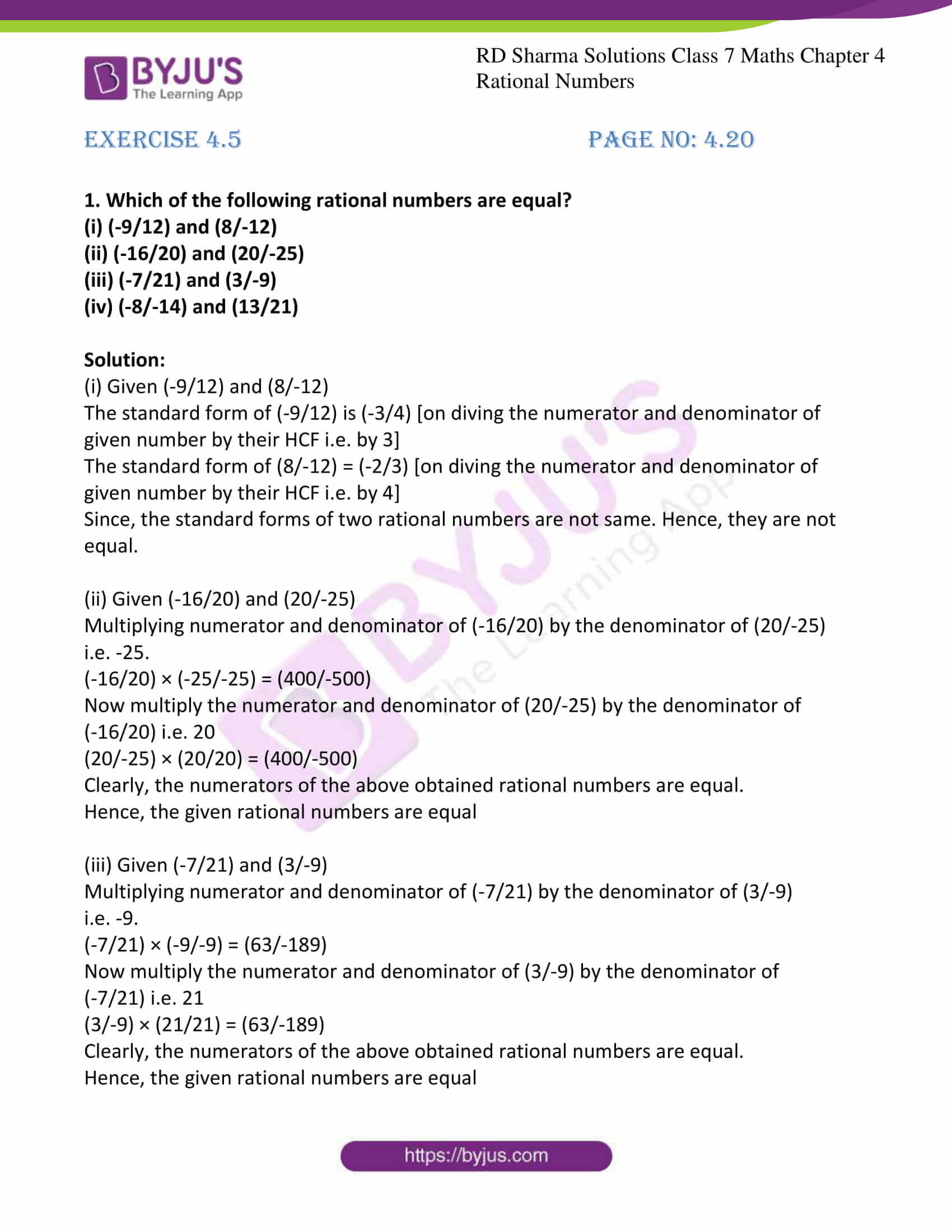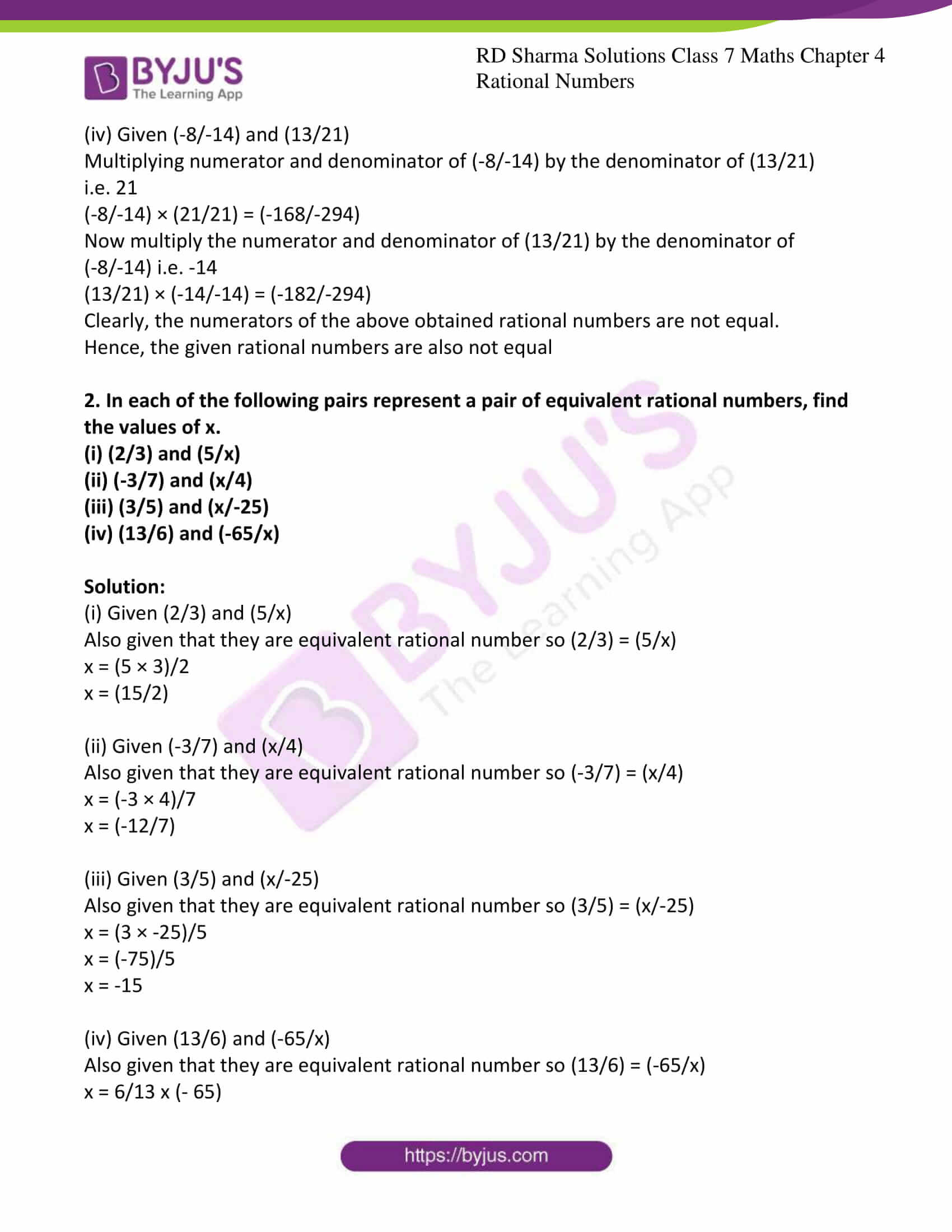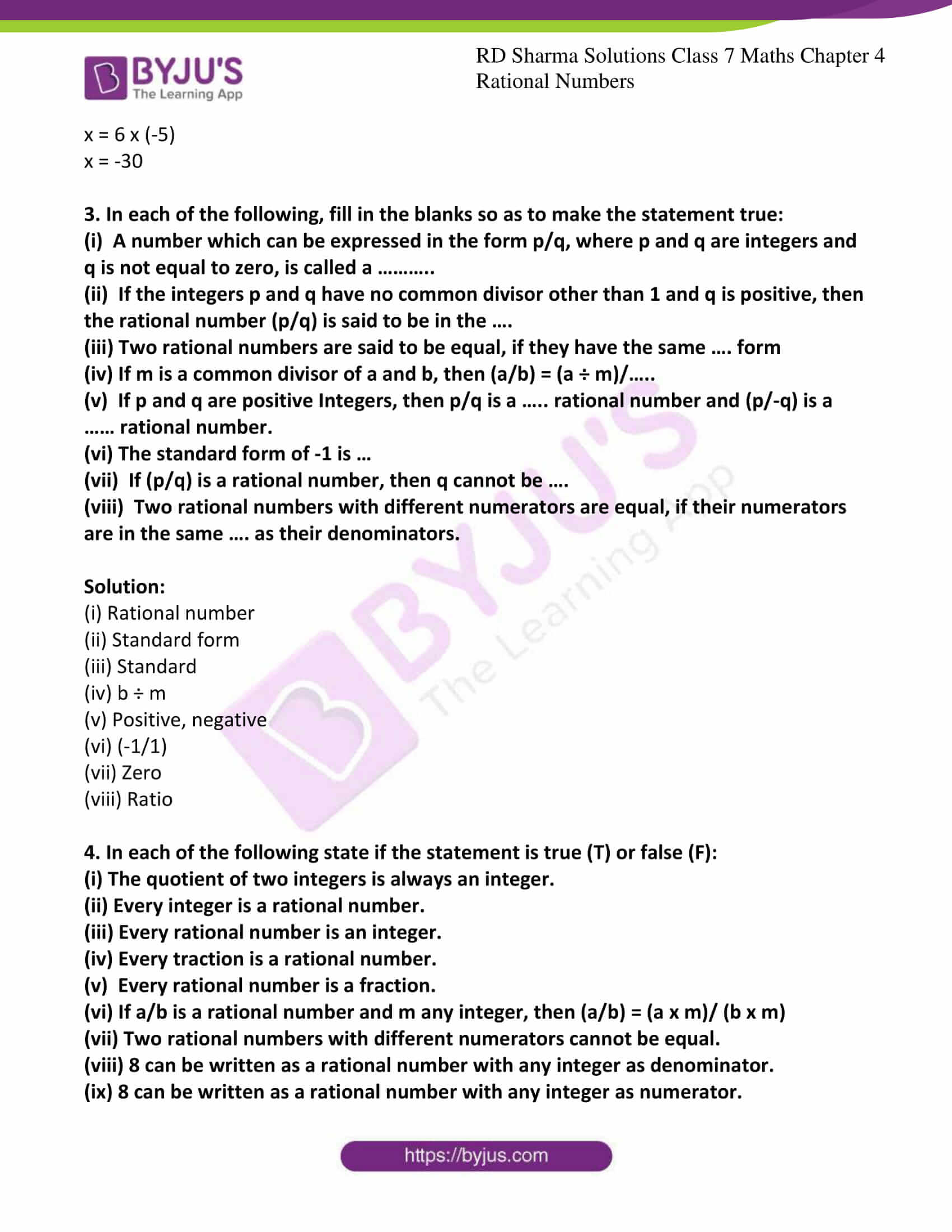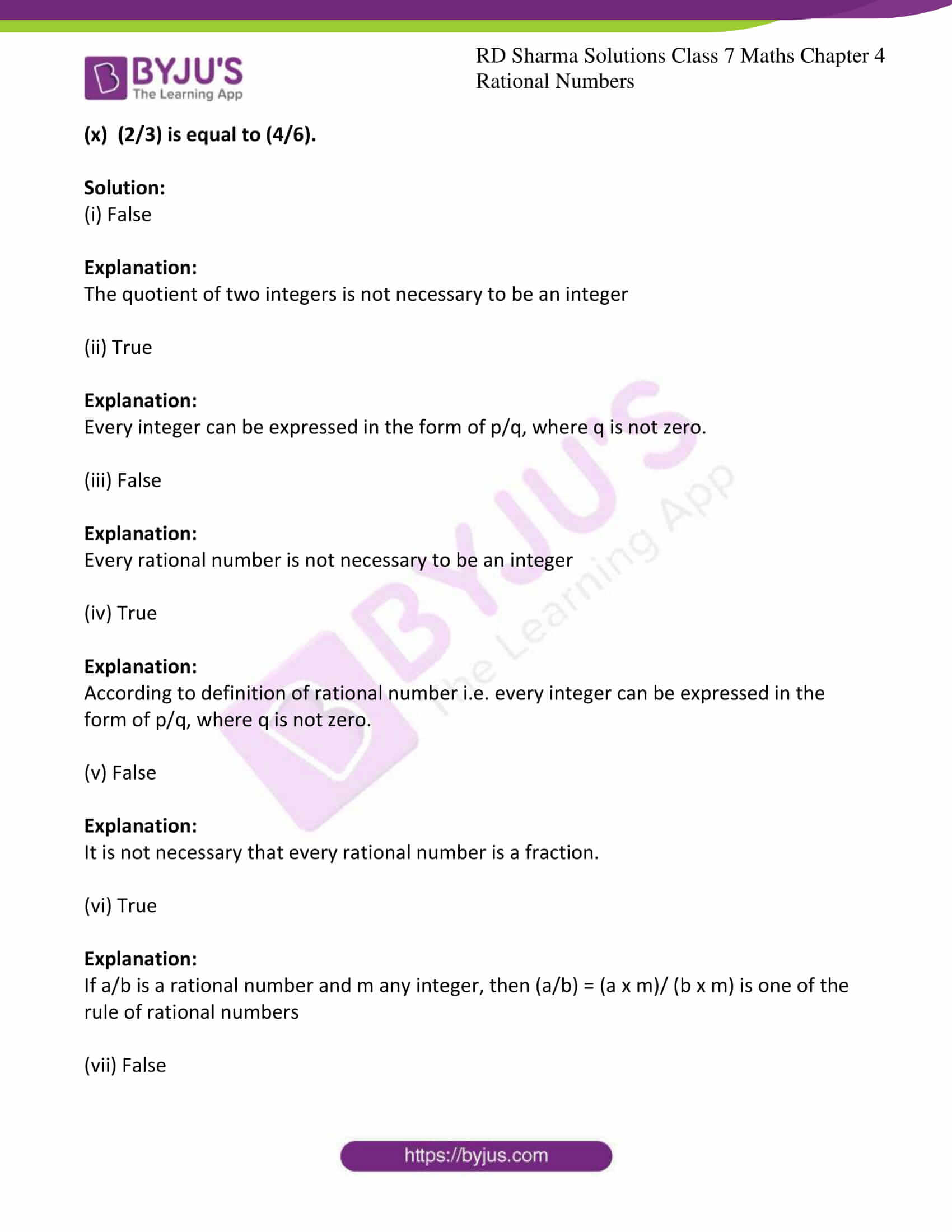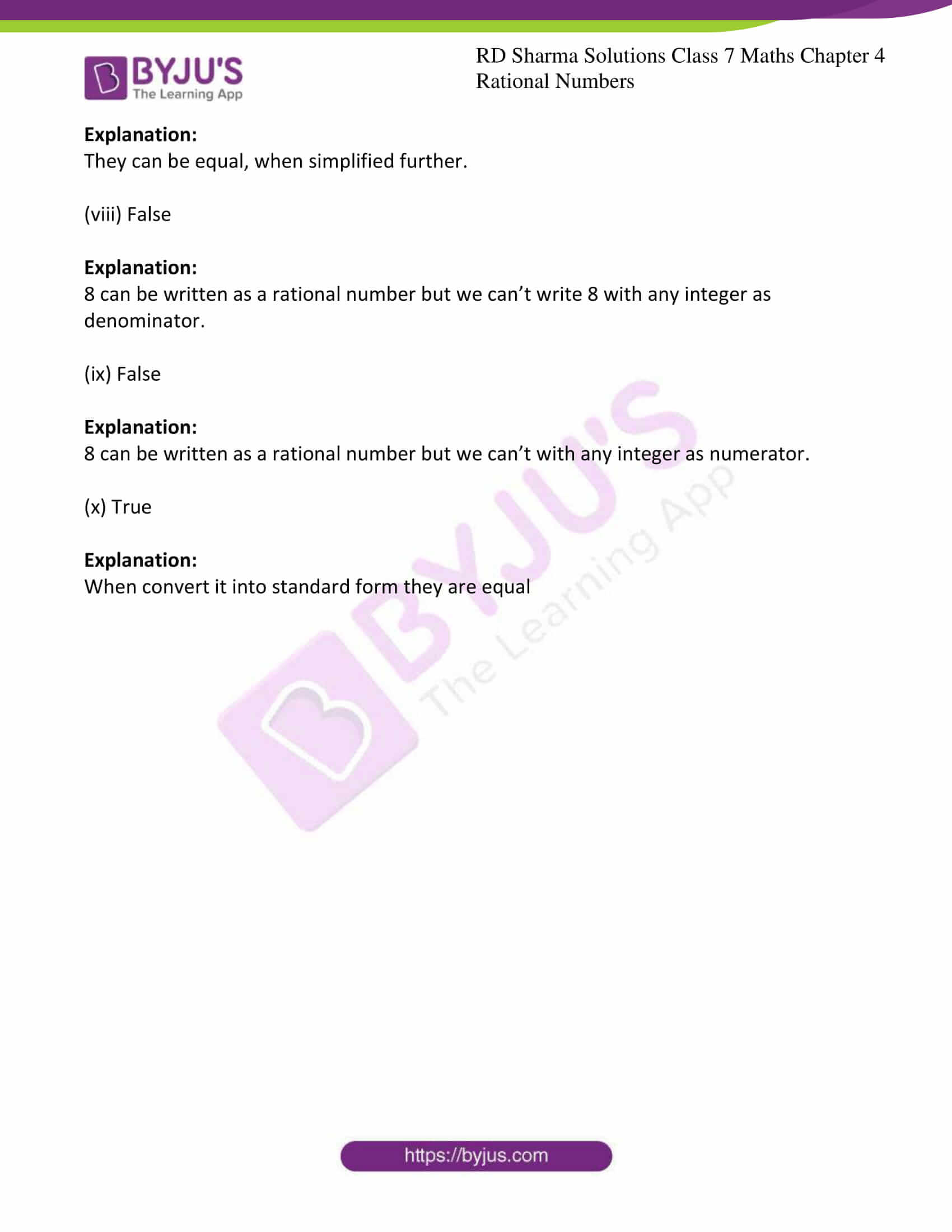### Access answers to Maths RD Sharma Solutions For Class 7 Chapter 4 – Rational Numbers Exercise 4.5

1. Which of the following rational numbers are equal?

(i) (-9/12) and (8/-12)

(ii) (-16/20) and (20/-25)

(iii) (-7/21) and (3/-9)

(iv) (-8/-14) and (13/21)

Solution:

(i) Given (-9/12) and (8/-12)

The standard form of (-9/12) is (-3/4) [on diving the numerator and denominator of given number by their HCF i.e. by 3]

The standard form of (8/-12) = (-2/3) [on diving the numerator and denominator of given number by their HCF i.e. by 4]

Since, the standard forms of two rational numbers are not same. Hence, they are not equal.

(ii) Given (-16/20) and (20/-25)

Multiplying numerator and denominator of (-16/20) by the denominator of (20/-25)

i.e. -25.

(-16/20) × (-25/-25) = (400/-500)

Now multiply the numerator and denominator of (20/-25) by the denominator of

(-16/20) i.e. 20

(20/-25) × (20/20) = (400/-500)

Clearly, the numerators of the above obtained rational numbers are equal.

Hence, the given rational numbers are equal

(iii) Given (-7/21) and (3/-9)

Multiplying numerator and denominator of (-7/21) by the denominator of (3/-9)

i.e. -9.

(-7/21) × (-9/-9) = (63/-189)

Now multiply the numerator and denominator of (3/-9) by the denominator of

(-7/21) i.e. 21

(3/-9) × (21/21) = (63/-189)

Clearly, the numerators of the above obtained rational numbers are equal.

Hence, the given rational numbers are equal

(iv) Given (-8/-14) and (13/21)

Multiplying numerator and denominator of (-8/-14) by the denominator of (13/21)

i.e. 21

(-8/-14) × (21/21) = (-168/-294)

Now multiply the numerator and denominator of (13/21) by the denominator of

(-8/-14) i.e. -14

(13/21) × (-14/-14) = (-182/-294)

Clearly, the numerators of the above obtained rational numbers are not equal.

Hence, the given rational numbers are also not equal

2. In each of the following pairs represent a pair of equivalent rational numbers, find the values of x.

(i) (2/3) and (5/x)

(ii) (-3/7) and (x/4)

(iii) (3/5) and (x/-25)

(iv) (13/6) and (-65/x)

Solution:

(i) Given (2/3) and (5/x)

Also given that they are equivalent rational number so (2/3) = (5/x)

x = (5 × 3)/2

x = (15/2)

(ii) Given (-3/7) and (x/4)

Also given that they are equivalent rational number so (-3/7) = (x/4)

x = (-3 × 4)/7

x = (-12/7)

(iii) Given (3/5) and (x/-25)

Also given that they are equivalent rational number so (3/5) = (x/-25)

x = (3 × -25)/5

x = (-75)/5

x = -15

(iv) Given (13/6) and (-65/x)

Also given that they are equivalent rational number so (13/6) = (-65/x)

x = 6/13 x (- 65)

x = 6 x (-5)

x = -30

3. In each of the following, fill in the blanks so as to make the statement true:

(i)  A number which can be expressed in the form p/q, where p and q are integers and q is not equal to zero, is called a ………..

(ii)  If the integers p and q have no common divisor other than 1 and q is positive, then the rational number (p/q) is said to be in the ….

(iii) Two rational numbers are said to be equal, if they have the same …. form

(iv) If m is a common divisor of a and b, then (a/b) = (a ÷ m)/…..

(v)  If p and q are positive Integers, then p/q is a ….. rational number and (p/-q) is a …… rational number.

(vi) The standard form of -1 is …

(vii)  If (p/q) is a rational number, then q cannot be ….

(viii)  Two rational numbers with different numerators are equal, if their numerators are in the same …. as their denominators.

Solution:

(i) Rational number

(ii) Standard form

(iii) Standard

(iv) b ÷ m

(v) Positive, negative

(vi) (-1/1)

(vii) Zero

(viii) Ratio

4. In each of the following state if the statement is true (T) or false (F):

(i) The quotient of two integers is always an integer.

(ii) Every integer is a rational number.

(iii) Every rational number is an integer.

(iv) Every traction is a rational number.

(v)  Every rational number is a fraction.

(vi) If a/b is a rational number and m any integer, then (a/b) = (a x m)/ (b x m)

(vii) Two rational numbers with different numerators cannot be equal.

(viii) 8 can be written as a rational number with any integer as denominator.

(ix) 8 can be written as a rational number with any integer as numerator.

(x)  (2/3) is equal to (4/6).

Solution:

(i) False

Explanation:

The quotient of two integers is not necessary to be an integer

(ii) True

Explanation:

Every integer can be expressed in the form of p/q, where q is not zero.

(iii) False

Explanation:

Every rational number is not necessary to be an integer

(iv) True

Explanation:

According to definition of rational number i.e. every integer can be expressed in the form of p/q, where q is not zero.

(v) False

Explanation:

It is not necessary that every rational number is a fraction.

(vi) True

Explanation:

If a/b is a rational number and m any integer, then (a/b) = (a x m)/ (b x m) is one of the rule of rational numbers

(vii) False

Explanation:

They can be equal, when simplified further.

(viii) False

Explanation:

8 can be written as a rational number but we can’t write 8 with any integer as denominator.

(ix) False

Explanation:

8 can be written as a rational number but we can’t with any integer as numerator.

(x) True

Explanation:

When convert it into standard form they are equal